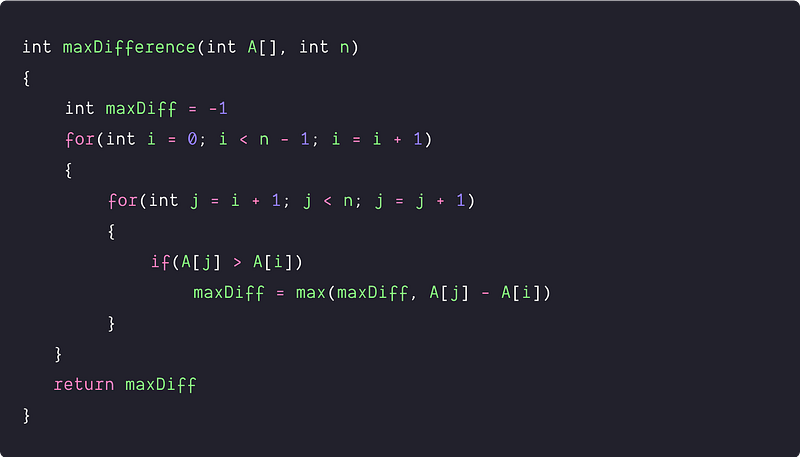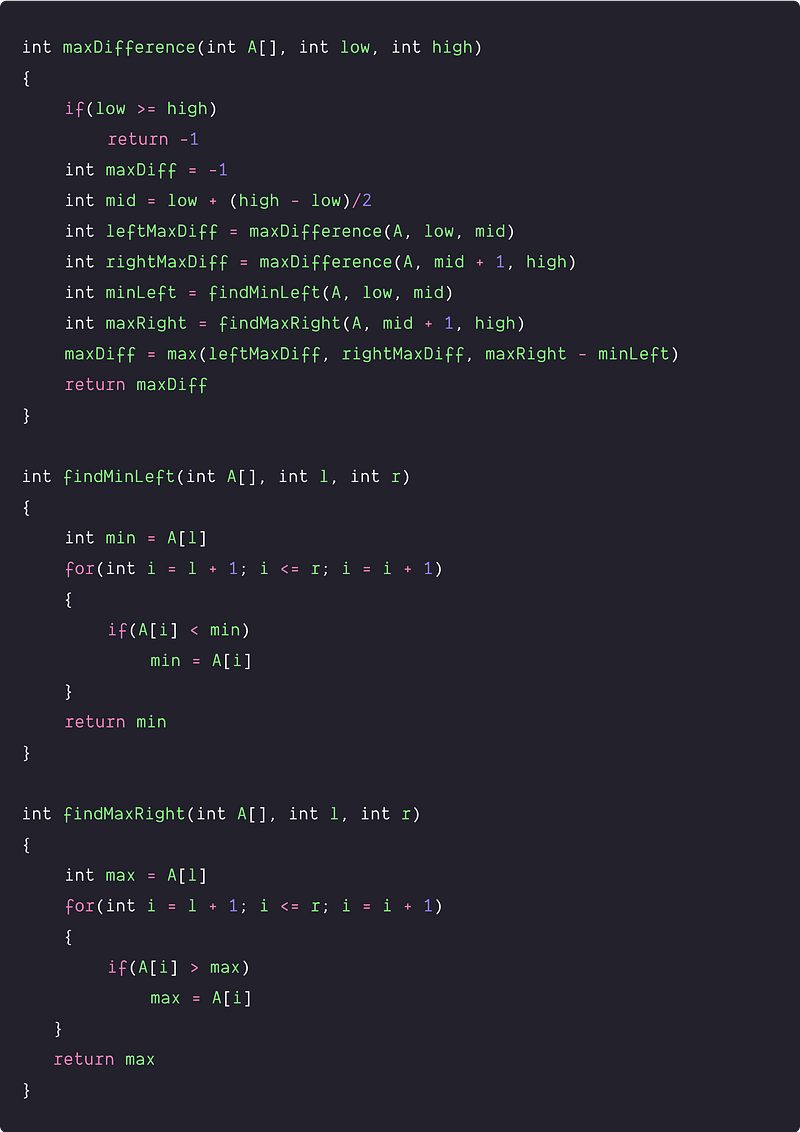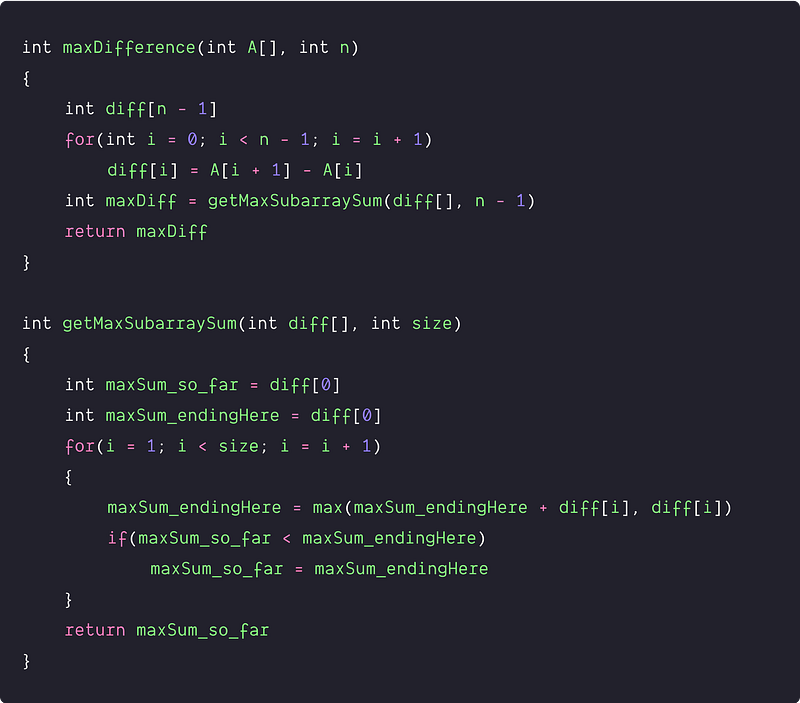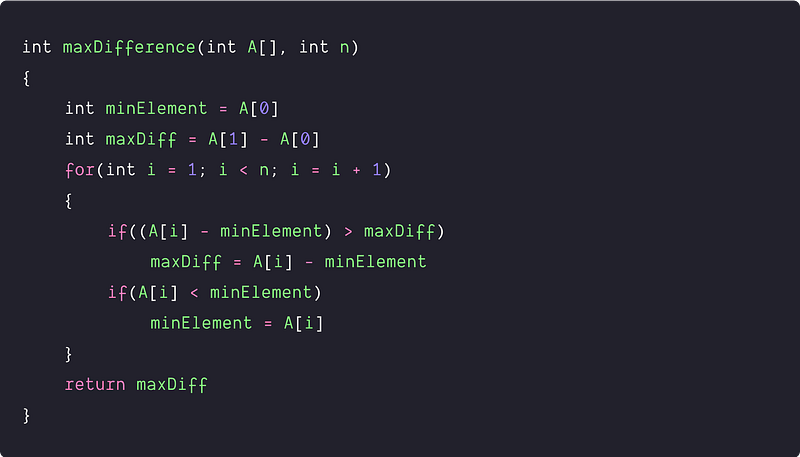# The maximum difference between two elements

Key takeaway: An excellent coding problem to learn problem-solving using divide and conquer, transform and conquer and a single loop.

### Let’s understand the problem

Given an array A[] of integers, write a program to find the maximum difference between any two elements such that the larger element appears after the smaller element. In other words, we need to find max(A[j] - A[i]), where A[j] > A[i] and j > i.

Example 1

Input: A[] = [1, 4, 9, 5, 3, 7], Output: 8

Explanation: Maximum difference is between 9 and 1.

Example 2

Input : A[] = [9, 8, 1, 6, 3, 2], Output : 5

Explanation: Maximum difference is between 6 and 1.

### Discussed solution approaches

• A brute force approach using nested loops
• Using divide and conquer approach similar to the merge sort
• Using transform and conquer approach: transforming the problem into max subarray sum problem using extra space
• An efficient in-place approach  using a single loop

### A brute force approach  using nested loops

Solution Idea

The basic idea is to explore each pair (A[j], A[i]) and find the maximum difference, where A[j] > A[i] and j > i. How do we implement this? Let's think!

We can use two nested loops where we pick each element from i = 0 to n-2 using the outer loop and find the difference with every element from j = i+1 to n-1 using an inner loop. We also keep track of the maximum difference where the larger element appears after the smaller element. Note: we exclude the last element in the outer loop because we need at least two elements for calculating the max difference.

Solution Steps

• Initialize a variable maxDiff to store the value of maximum difference.
• Now we run an outer loop from i = 0 to n-2
• For every element A[i], we run an inner loop from i+1 to n-1. Whenever we find A[j] > A[i], we update the maxDiff with max(A[j] - A[i], maxDiff).
• By the end of the nested loops, we return maxDiff.

Solution PseudocodeTime and space complexity analysis

We are running nested loops and exploring each unique pair (A[j], A[i]). So total number of loop iteration = total count of unique pairs = nC2 = n(n-1)/2. At each iteration, we are performing O(1) operation. So time complexity = n(n-1)/2 * O(1) = O(n^2).

We are using a constant number of extra variables, so space complexity = O(1).

### Divide and conquer approach similar to the merge sort

Solution Idea

Can we solve this problem using the divide and conquer approach or using the solution of the smaller sub-problems? Let's think!

Suppose we divided the array into two equal parts and calculated the max difference of the left and right parts recursively. Then the max diff of the overall array will be the maximum of these three possibilities :

• max difference of the left part (Subproblem 1)
• max difference of the right part(Subproblem 2)
• max difference crossing the left and the right part. This is a situation when the min value is from the left part, the max value is from the right part (Think!)

So, the max difference = max (max difference of the left part, max difference of the right part, max difference crossing the left and right part). This idea looks similar to the merge sort or divide conquer solution of the maximum sub-array sum. (Think!)

Solution Steps

Divide part: Divide the array into two equal halves, mid = l + (r  -  l)/2

Conquer part: Recursively solve the smaller sub-problems.

• leftMaxDiff = maxDifference (A[], low, mid),
• rightMaxDiff = maxDifference (A[], mid + 1, right)

Combine part: We calculate the max diff crossing the left and right parts.

• Find minimum from the left part i.e. minLeft = findMinLeft(A[], low, mid)
• Find maximum from the right part i.e maxRight = findMaxRight(A[], mid + 1, high).
• So, the max diff crossing the left and right part = maxRight  -  minLeft (Think!)

So, overall max difference will be the maximum of leftMaxDiff, rightMaxDiff and maxRight- minLeft i.e. maxDiff = max(leftMaxDiff, rightMaxDiff, maxRight- minLeft).

Base case: low≥high would be the base case which is a scenario of the single element. The idea is simple: for calculating the max difference, we need at least two elements in the array.

Solution PseudocodeTime and space complexity analysis

For a recursive solution, we need to write down the recurrence relation to calculate the time complexity. Suppose T(n) is the time complexity of finding the max difference for input size n.

So T(n) = Time complexity of the divide part + Time complexity of the conquer part + Time complexity of the combine part

• The time complexity of the divide part = O(1) (Think!)
• The time complexity of the conquer part = 2T(n/2) because we are recursively solving two sub-problems, each of size n/2.
• The time complexity of the combine part = time complexity of finding min in the left part + time complexity of finding max in the right part + calculating max difference = O(n) + O(n) + O(1) = O(n)

After adding all three-time complexities, we will get the recurrence relation for the overall time complexity.

T(n) = O(1) + 2 T(n/2) + O(n) = 2 T(n/2) + cn.

In more general words

• T (n) = c, if n = 1
• T(n) = 2 T(n/2) + cn, if n > 1

This recurrence looks similar to the merge sort recurrence, so we can solve it using the recursion tree method or master theorem. Hence, Time complexity = O(nlogn)

For the space complexity of the recursive program, we need to calculate the size of the recursion call stack. The size of the recursion call stack = height of the recursion tree = O(logn). Space complexity: O(logn) (Think!)

### Using transform and conquer approach: transforming the problem into max subarray sum problem using extra space

Solution Idea

The main idea behind this algorithm is to go through the difference between adjacent elements and store all differences in an auxiliary array of size n-1. Now, this problem gets transformed into finding the maximum sum subarray of this difference array. How? Think!

Suppose for some j and i, we can write A[j] - A[i] in terms of sum of the difference between adjacent elements from i to j. Here are the steps:

=> A[j] - A[i] = (A[j] - A[j-1]) + (A[j-1] - A[j-2]) + ...... + (A[i+1] - A[i])

So, max (A[j] - A[i]) = max [(A[j] - A[j-1]) + (A[j-1] - A[j-2]) + ...... + (A[i+1] - A[i])]

=> max (A[j] - A[i]) = max [diff (j, j-1) + diff (j-1, j-2) +..... + diff (i+1, i)]

In other words, max (A[j] - A[i]) = max (subarray sum of the differences from index i to j)

So from the above observations, if we store the differences of the adjacent elements in an extra array diff[], then we can easily calculate the max (A[j] - A[i]) by finding the max subarray sum of the diff[] array. Note: the size of the difference array would be n-1. Think!

Solution Steps

• Declare an extra memory diff[n-1] of size n-1 to store the differences of the adjacent elements from index 0 to n-1.
• We run a loop from 0 to n-2 to store the differences in diff[n-1].

``````for(int i = 0; i < n - 1; i = i + 1)
diff[i] = A[i + 1] - A[i]
``````
• Now we calculate and return the maximum subarray sum of the diff[] array. We can find this value in O(n) time and O(1) space. Refer to the finding max subarray sum blog.

Solution PseudocodeTime and Space complexity analysis

Time complexity = Time complexity of storing values in the diff[] array + Time complexity of finding max subarray sum of the diff[] array = O(n) + O(n) = O(n)

Space complexity = O(n), for storing the differences in diff[] array of size n-1.

### An efficient in-place approach  using a single loop

Solution Idea

In this method, instead of taking the difference of each element with every other element, we take the difference with the smallest element found so far. So we need to keep track of two things:

• The maximum difference found so far.
• The minimum element visited so far.

Solution Steps

• Traverse the array from left to right.
• Maintain two variables : minElement to store the smallest element found so far and maxDiff to store maximum difference so far.
• At every iteration, keep updating the minElement and maxDiff.
• By end of the loop, we return the value maxDiff.

Solution PseudocodeTime and space complexity analysis

We are running a single loop n-1 times and doing O(1) operations at each iteration of the loop. So the time complexity = (n-1) * O(1) = O(n). Space complexity = O(1), as we are using a constant number of extra variables.

### Critical ideas to think!

• In the divide and conquer approach : why are we considering the three different cases? Why space complexity is O(logn)?
• What would be the best and worst-case scenario of all the above approaches?
• In the transform and conquer approach : why are we calculating the max subarray sum of the diff[] array? can we modify this approach to solve the problem using constant extra space?
• How do the above solutions work in the case of repeating elements? do we need to modify the code?
• can solve this problem using a different approach? Think!

### Comparisons of time and space complexities

• Using two nested loops: Time = O(n^2), space = O(1)
• Using divide and conquer: Time = O(nlogn), space = O(logn)
• Using transform and conquer: Time = O(n), space = O(n)
• Using single scan: Time = O(n), space = O(1)

### Suggested coding problems to practice

Enjoy learning, Enjoy algorithms!

Get well-designed application and interview centirc content on ds-algorithms, machine learning, system design and oops. Content will be delivered weekly.